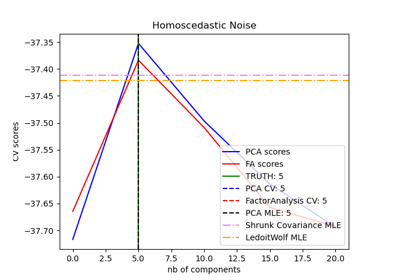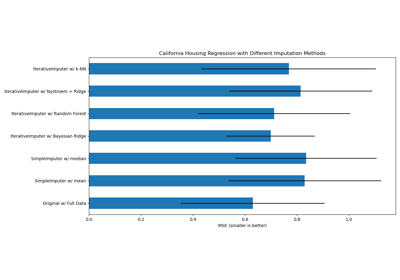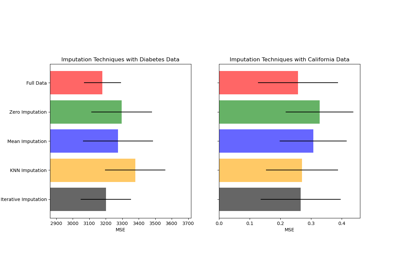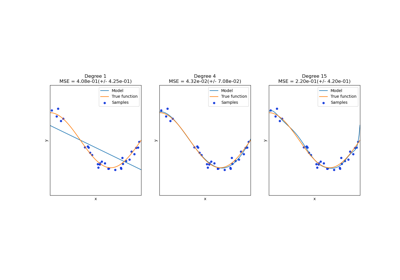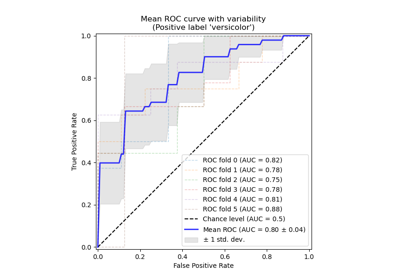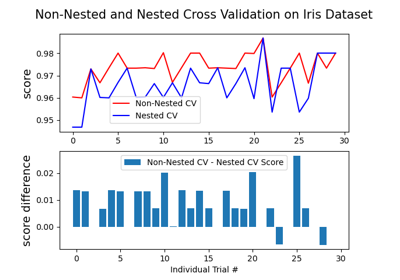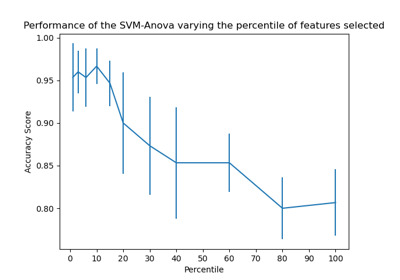# sklearn.model_selection.cross_val_score¶

sklearn.model_selection.cross_val_score(estimator, X, y=None, *, groups=None, scoring=None, cv=None, n_jobs=None, verbose=0, fit_params=None, pre_dispatch='2*n_jobs', error_score=nan)[source]

Evaluate a score by cross-validation

Read more in the User Guide.

Parameters
estimatorestimator object implementing ‘fit’

The object to use to fit the data.

Xarray-like of shape (n_samples, n_features)

The data to fit. Can be for example a list, or an array.

yarray-like of shape (n_samples,) or (n_samples, n_outputs), default=None

The target variable to try to predict in the case of supervised learning.

groupsarray-like of shape (n_samples,), default=None

Group labels for the samples used while splitting the dataset into train/test set. Only used in conjunction with a “Group” cv instance (e.g., GroupKFold).

scoringstr or callable, default=None

A str (see model evaluation documentation) or a scorer callable object / function with signature scorer(estimator, X, y) which should return only a single value.

Similar to cross_validate but only a single metric is permitted.

If None, the estimator’s default scorer (if available) is used.

cvint, cross-validation generator or an iterable, default=None

Determines the cross-validation splitting strategy. Possible inputs for cv are:

• None, to use the default 5-fold cross validation,

• int, to specify the number of folds in a (Stratified)KFold,

• An iterable yielding (train, test) splits as arrays of indices.

For int/None inputs, if the estimator is a classifier and y is either binary or multiclass, StratifiedKFold is used. In all other cases, KFold is used.

Refer User Guide for the various cross-validation strategies that can be used here.

Changed in version 0.22: cv default value if None changed from 3-fold to 5-fold.

n_jobsint, default=None

Number of jobs to run in parallel. Training the estimator and computing the score are parallelized over the cross-validation splits. None means 1 unless in a joblib.parallel_backend context. -1 means using all processors. See Glossary for more details.

verboseint, default=0

The verbosity level.

fit_paramsdict, default=None

Parameters to pass to the fit method of the estimator.

pre_dispatchint or str, default=’2*n_jobs’

Controls the number of jobs that get dispatched during parallel execution. Reducing this number can be useful to avoid an explosion of memory consumption when more jobs get dispatched than CPUs can process. This parameter can be:

• None, in which case all the jobs are immediately created and spawned. Use this for lightweight and fast-running jobs, to avoid delays due to on-demand spawning of the jobs

• An int, giving the exact number of total jobs that are spawned

• A str, giving an expression as a function of n_jobs, as in ‘2*n_jobs’

error_score‘raise’ or numeric, default=np.nan

Value to assign to the score if an error occurs in estimator fitting. If set to ‘raise’, the error is raised. If a numeric value is given, FitFailedWarning is raised.

New in version 0.20.

Returns
scoresndarray of float of shape=(len(list(cv)),)

Array of scores of the estimator for each run of the cross validation.

cross_validate

To run cross-validation on multiple metrics and also to return train scores, fit times and score times.

cross_val_predict

Get predictions from each split of cross-validation for diagnostic purposes.

sklearn.metrics.make_scorer

Make a scorer from a performance metric or loss function.

Examples

>>> from sklearn import datasets, linear_model
>>> from sklearn.model_selection import cross_val_score
>>> X = diabetes.data[:150]
>>> y = diabetes.target[:150]
>>> lasso = linear_model.Lasso()
>>> print(cross_val_score(lasso, X, y, cv=3))
[0.33150734 0.08022311 0.03531764]


## Examples using sklearn.model_selection.cross_val_score¶